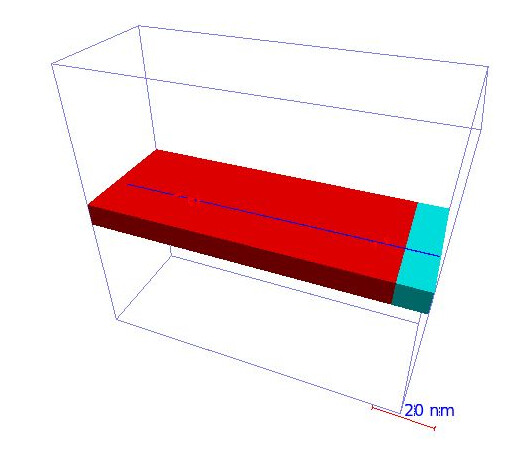# Using the G4EmCalculator to get stopping power for a layered device structure

Hello,
I have been working on the extended Geant4 example TestEm0 that uses G4EmCal to obtain stopping power for a given material. The original geometry comprises a cube of only a single material. I built up on that by creating a layered structure of different materials, similar to the below (layer 1: blue region, Si; layer 2: red region, SiO2).So far, I get a value of stopping power each for layer 1 and layer 2 both at 10 MeV, in accordance to the NIST database.I use an incident proton beam of, say 10 MeV. G4EmCal is deployed once for each material.

// get unrestricted Stopping power for second material
std::vector dedx11;
std::vector dedx21;
G4double dedx_1, dedxtot_1 = 0.;
size_t nproc1 = emName.size(); //emName accounts for process lists and extracts em processes
for (size_t j=0; j<nproc1; j++) {
dedx_1= emCal.ComputeDEDX(energy,particle,emName[j],material1,energy);
dedxtot_1 += dedx_1;
dedx11[j] = dedx_1;
dedx21[j] = dedx_1/density1;
}
dedx11[nproc1] = dedxtot_1;
dedx21[nproc1] = dedxtot_1/density1;
//print stopping power
G4cout << "\n \n unrestricted dE/dx for second material : ";
for (size_t j=0; j<=nproc1; j++) {
G4cout << “\t” << std::setw(13) << G4BestUnit(dedx11[j],“Energy/Length”);
}

However, I am interested in the stopping power of layer 1 at 10 MeV and of layer 2 at whatever incident energy would be lost from layer 1. How can I account for the change of energy from one layer to the next and obtain the stopping power accordingly, ideally using G4EmCal?